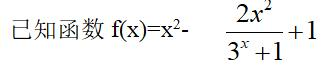# 功能整体对等与部分对等

.若存在m∈(1,4)使得不等式f(4-ma) f(m2 3m)>2成立，则实数a的取值范围为四个城市 苏锡场镇2020~2021学年高三教学情况调查（二）

A.(-∞,7) B.(-∞,7] C.( -∞,8) D.(-∞,8]g(x)=1 x sinx partial parity

A[-2,2] B [-1,1] C[0,2] D[1,3]

f(1)≤f(x-1)≤f(-1)

F(x) f(-x)=g(X) 1 g(-x ) 1=2